Question

# Compute the reliability of a system with 7 components, neading with at least 2 in full...

Compute the reliability of a system with 7 components, neading with at least 2 in full operation with the MTTF of each component being 2100 hours, after 2510 hours of operation

mean = 2100

X = lifetime of a system

X follow exponential distribution with mean = 2100

P(X > 2510) = e^(-2510/2100)= 0.30263

Y = number of component in full operation

Y follow binomial distribution with n = 7 , p = 0.30263

P(Y >= 2 )

=binom.dist.range(7, 0.30263,2,7)

= 0.672612

#### Earn Coins

Coins can be redeemed for fabulous gifts.

Similar Homework Help Questions
• ### Compute the reliability of a system with 5 components in standby parallel configuration, with failure rate...

Compute the reliability of a system with 5 components in standby parallel configuration, with failure rate of 0.000208after 4300 hours of operation Correct Answer: 0.997717521

• ### module, consists of five repairable components, all of which must operate for system success. Each component performs a different function but all five share identical reliability parameters. Spe...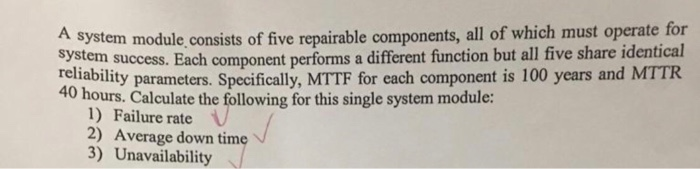module, consists of five repairable components, all of which must operate for system success. Each component performs a different function but all five share identical reliability parameters. Specifically, MTTF for each component is 100 years and MTTR 40 hours. Calculate the following for this single system module: 1) Failure rate 2) Average down time 3) Unavailability module, consists of five repairable components, all of which must operate for system success. Each component performs a different function but all five share...

• ### 2. Consider the reliability model given in Assignment 5 Problem 1. You have discovered that the...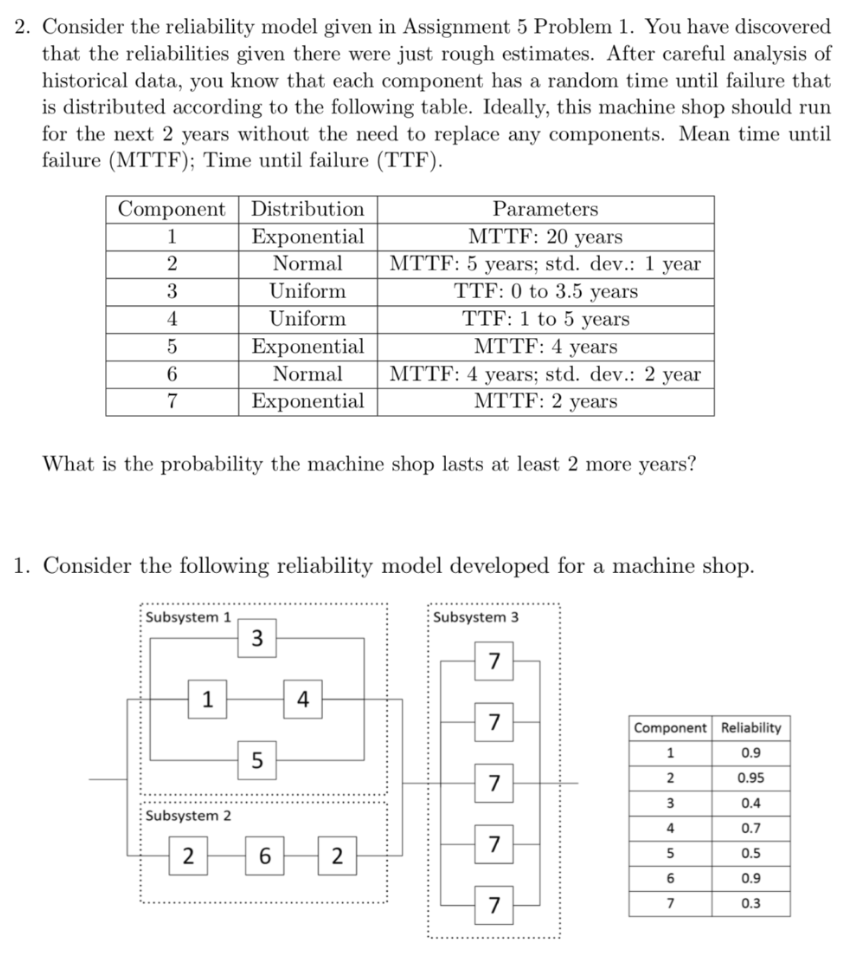2. Consider the reliability model given in Assignment 5 Problem 1. You have discovered that the reliabilities given there were just rough estimates. After careful analysis of historical data, you know that each component has a random time until failure that is distributed according to the following table. Ideally, this machine shop should run for the next 2 years without the need to replace any components. Mean time until failure (MTTF); Time until failure (TTF) Component Distribution Exponential Parameters MTTF:...

• ### I want help to solve this question A system is made up of three independent components....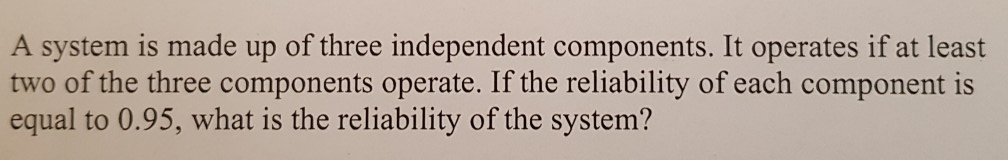I want help to solve this question A system is made up of three independent components. It operates if at least two of the three components operate. If the reliability of each component is equal to 0.95, what is the reliability of the system?

• ### 4. Reliability of Systems - Take n components to have failure times Ti, T2, ..., Tn...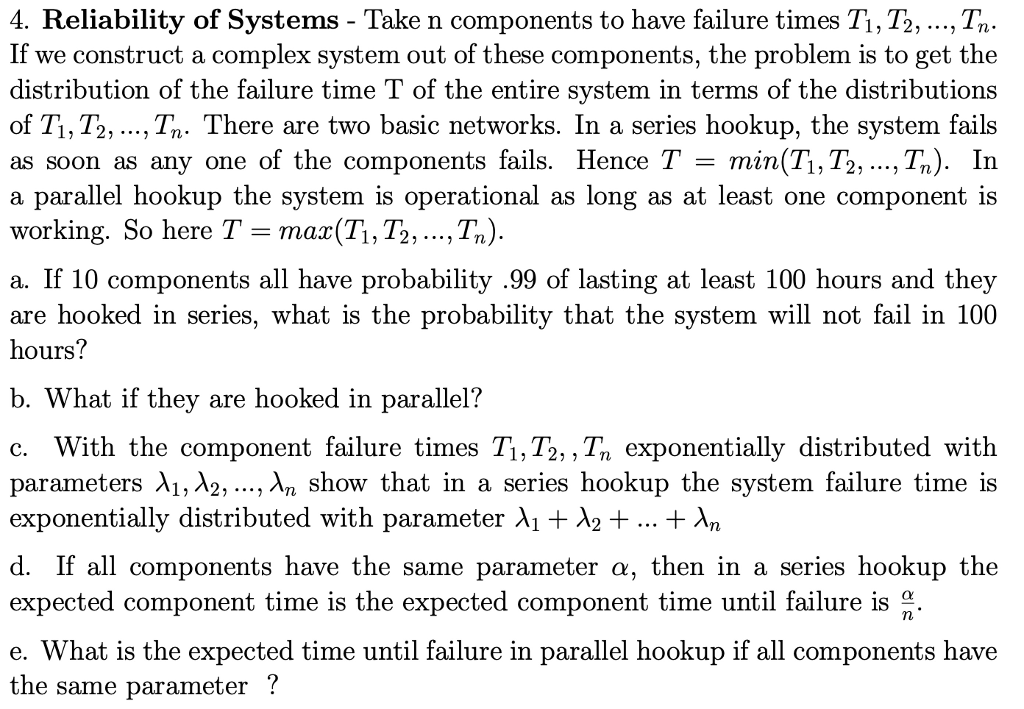4. Reliability of Systems - Take n components to have failure times Ti, T2, ..., Tn If we construct a complex system out of these distribution of the failure time T of the entire svstem in terms of the distributions of Ti, T2, ..., Tn. There are two basic networks. In a series hookup, the system fails as soon as any one of the components fails. Hence T - min(T1, T2, ...,Tn). In a parallel hookup the system is operational...

• ### Question 3 20 pts A system has three components with reliability values A, B, and C....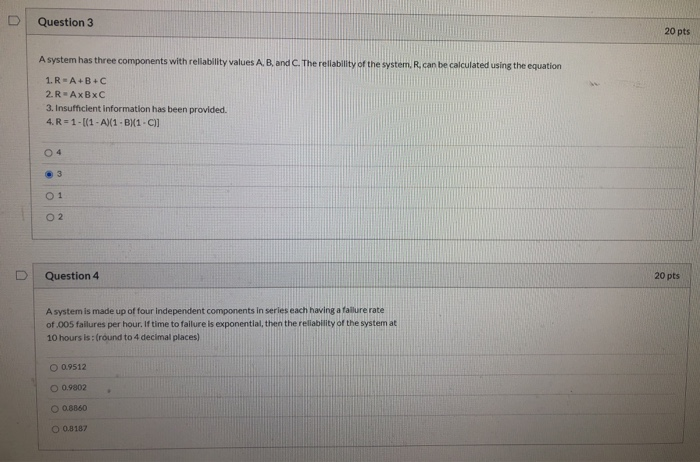Question 3 20 pts A system has three components with reliability values A, B, and C. The reliability of the system. R. can be calculated using the equation 1.R - A+B+C 2.R-AxBxC 3. Insufficient Information has been provided. 4. R = 1 - (1 - A)(1-B1-C)] 04 O 2 U Question 4 20 pts A system is made up of four independent components in series each having a failure rate of .005 failures per hour. If time to failure is...

• ### A system module, consists of five repairable components, all of which must operate for System success. Each component performs a different function but all five share identical relhability parameters...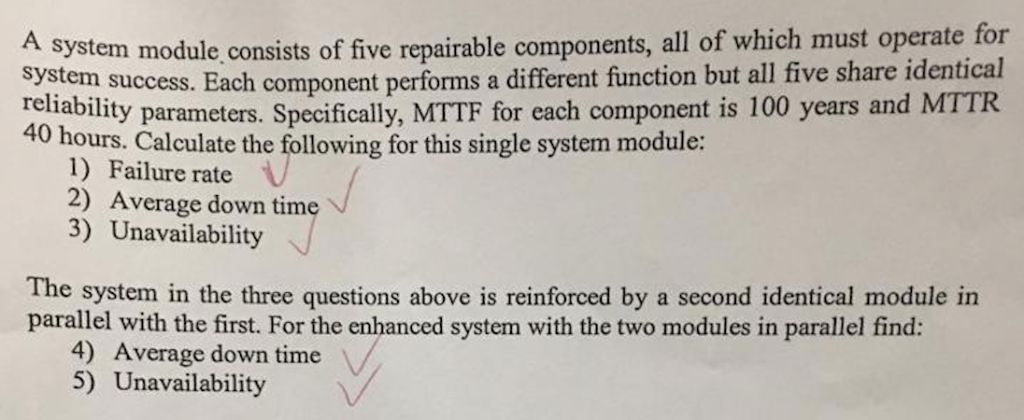A system module, consists of five repairable components, all of which must operate for System success. Each component performs a different function but all five share identical relhability parameters. Specifially, MTTF for each component is 100 years and MTTR 40 hours. Calculate the following for this single system module: 1) Failure rate V 2) Average down time 3) Unavailability The system in the three questions above is reinforced by a second identical module in parallel with the first. For the...

• ### A system module, consists of five repairable components, all of which must operate for System success. Each component performs a different function but all five share identical relhability parameters...A system module, consists of five repairable components, all of which must operate for System success. Each component performs a different function but all five share identical relhability parameters. Specifially, MTTF for each component is 100 years and MTTR 40 hours. Calculate the following for this single system module: 1) Failure rate V 2) Average down time 3) Unavailability The system in the three questions above is reinforced by a second identical module in parallel with the first. For the...

• ### Problem 4 Consider the system of components connected as depicted below. The system can be thought...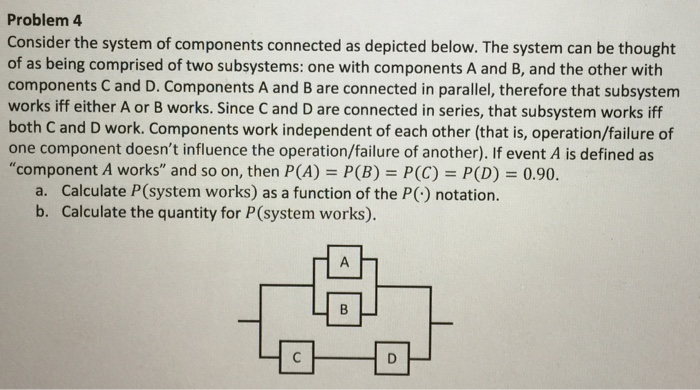Problem 4 Consider the system of components connected as depicted below. The system can be thought of as being comprised of two subsystems: one with components A and B, and the other with components C and D. Components A and B are connected in parallel, therefore that subsystem works iff either A or B works. Since C and D are connected in series, that subsystem works iff both C and D work. Components work independent of each other (that is,...

• ### 7 - 22 (page 365). Spacescope Inc. has an electronic component that has a failure rate...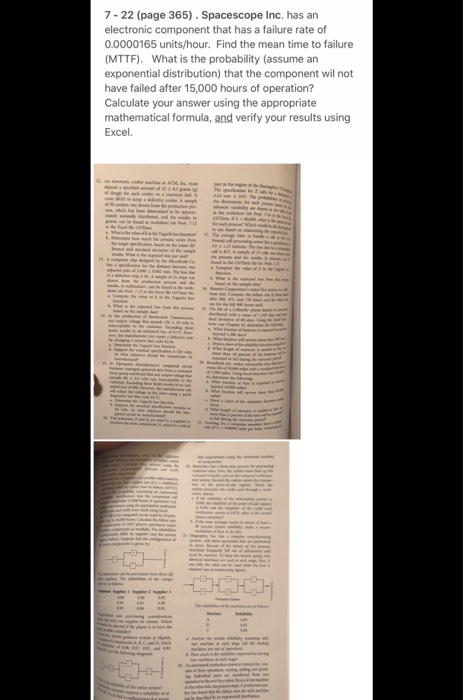7 - 22 (page 365). Spacescope Inc. has an electronic component that has a failure rate of 0.0000165 units/hour. Find the mean time to failure (MTTF). What is the probability (assume an exponential distribution) that the component wil not have failed after 15,000 hours of operation? Calculate your answer using the appropriate mathematical formula, and verify your results using Excel.